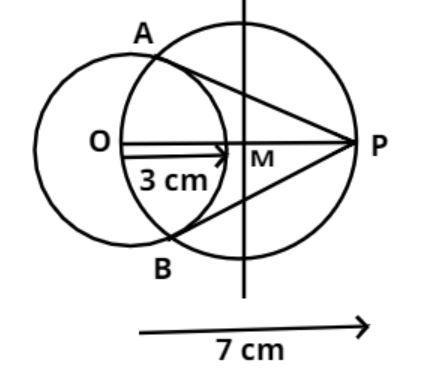QuestionAnswers

# Draw a circle of radius 3cm. Mark a point 7cm away from its center. Draw the tangents to the circle from this point.Verified
159.9k+ views
Hint: Start with drawing the first circle and use rounder to do the construction.Given:
$\left( 1 \right).$Draw a circle 3cm radius and O as its center. From center mark OP = 7cm,
$\left( 2 \right).$Draw perpendicular bisector of the line OP and meet at M.
$\left( 3 \right).$Draw a circle with radius $OM$and cut the circle at A and B.
$\left( 4 \right).$Joint PA and PB.